# II Review | Constants | Complete the following table. (All solutions are at 25 °C.) Solution...II Review | Constants | Complete the following table. (All solutions are at 25 °C.) Solution [H3O+] [OH-] pH Acidic, basic, or neutral Part A 1 3.5 x 10-3 Complete the first column for [H3O+] of Solutions 2 and 4. Express your answers to two significant figures separated by a comma. 2 3.8 x 10-7 3 1.8 x 10-9 ► View Available Hint(s) 4 7.15 IVALO ? [H3O+]2, [H3O+]4 = Submit Previous Answers X Incorrect; Try Again; 5 attempts remaining
Show transcribed image text

This Homework Help Question: "II Review | Constants | Complete the following table. (All solutions are at 25 °C.) Solution..." No answers yet.

We need 10 more requests to produce the answer to this homework help question. Share with your friends to get the answer faster!

0 /10 have requested the answer to this homework help question.

Once 10 people have made a request, the answer to this question will be available in 1-2 days.
All students who have requested the answer will be notified once they are available.
##### Add Answer of: II Review | Constants | Complete the following table. (All solutions are at 25 °C.) Solution...
Similar Homework Help Questions
• ### Review | Constants Periodic For each of the following solutions, calculate the initial pH and the...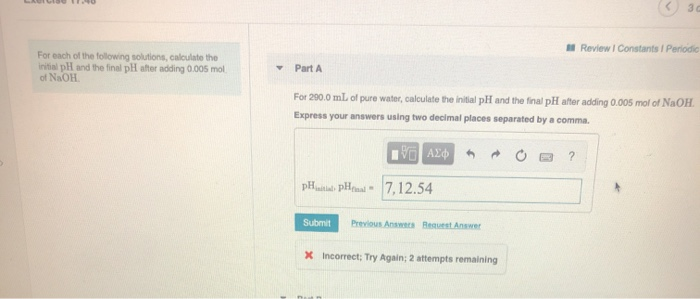Review | Constants Periodic For each of the following solutions, calculate the initial pH and the final pH after adding 0.005 mol of NaOH. Part A For 200.0 mL of pure water, calculate the initial pH and the final pH after adding 0.005 mol of NaOH. Express your answers using two decimal places separated by a comma. EVO AXO O ? pH penal - 7,12.54 Submit Previous A Request Answer X Incorrect; Try Again: 2 attempts remaining Review Constants Periodic...

• ### Question 5 ( 5 of 25 Review | Constants Periodic Table Complete the following table for...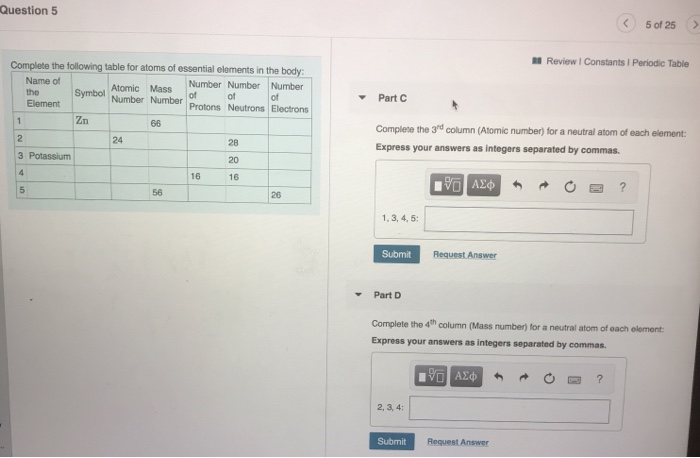Question 5 ( 5 of 25 Review | Constants Periodic Table Complete the following table for atoms of essential elements in the body: Name of Number Number Number the Symbol Atomic Mass "Number Number of of Element Protons Neutrons Electrons Zn of Part C Complete the 3rd column (Atomic number) for a neutral atom of each element: Express your answers as integers separated by commas. 3 Potassium % ALO O ? 1.3,4,5 Submit Request Answer - Part D Complete the...

• ### II Review | Constants | Periodic Table Part G For 4.8 x 10-4 M Ca(OH)2, determine...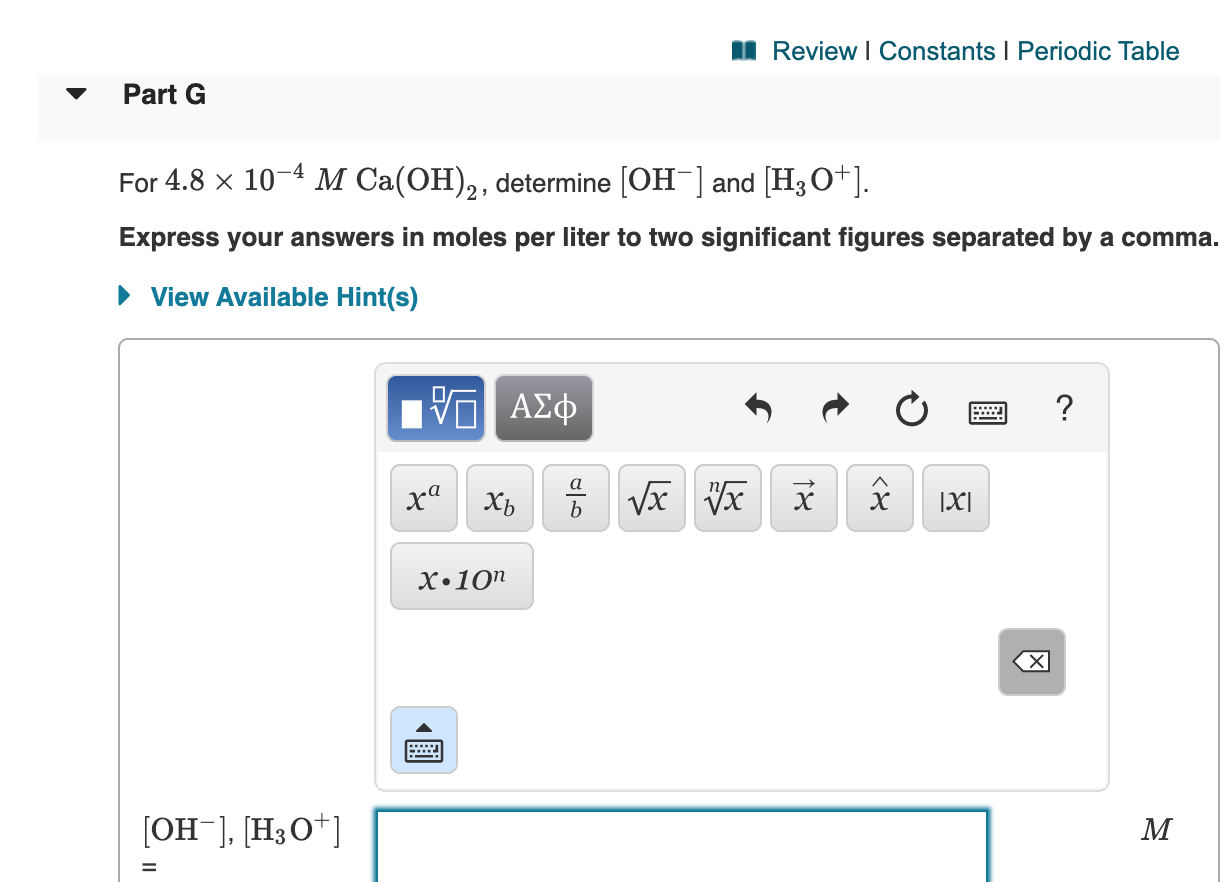II Review | Constants | Periodic Table Part G For 4.8 x 10-4 M Ca(OH)2, determine [OH-] and [H3O+]. Express your answers in moles per liter to two significant figures separated by a comma. ► View Available Hint(s) V AC X.10n x [OH-], [H30+] M M

• ### Chapter 04 Post-Lecture cuestion 5 < 5 of 25 Review Constants Periodic Ta Part A Atomic...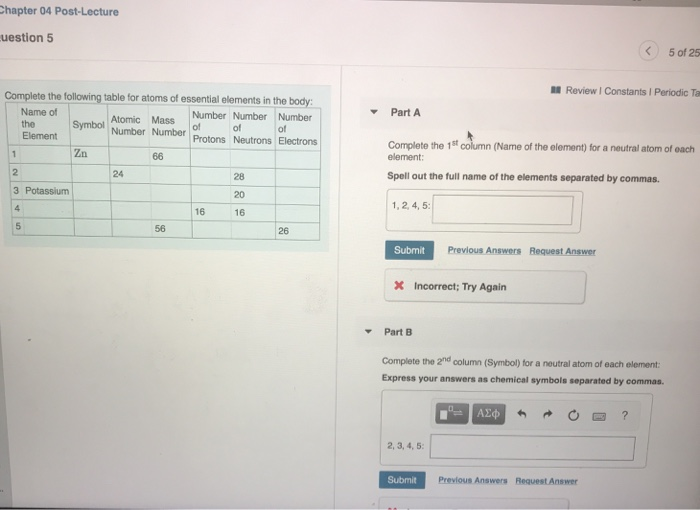Chapter 04 Post-Lecture cuestion 5 < 5 of 25 Review Constants Periodic Ta Part A Atomic Mass of of of Complete the following table for atoms of essential elements in the body: Name of Atomic Mass Number Number Number the Symbol Element Number Number Protons Neutrons Electrons Zn 66 24 28 3 Potassium Complete the 1st column (Name of the element) for a neutral atom of each element: Spell out the full name of the elements separated by commas. 20...

• ### help please Complete the following table. (The first row is completed for you.) [H3O+] [OH-] POH...help please Complete the following table. (The first row is completed for you.) [H3O+] [OH-] POH PH 1.0x10-4 1.0x10-10 10.00 4.00 Acidic or Basic acidic 8.5x10-3 4.2x10-6 6.8x10-9 7.65 Part A Complete the first column of the table. Express your answers using two significant figures. Enter your answers in order given in the table separated by a com ΟΙ ΑΣφ ? [H3O+] - M Submit Request Answer Part B Complete the second column of the table. Express your answers using...

• ### A Review | Constants Periodic Complete and balance each of the following equations for combustion reactions....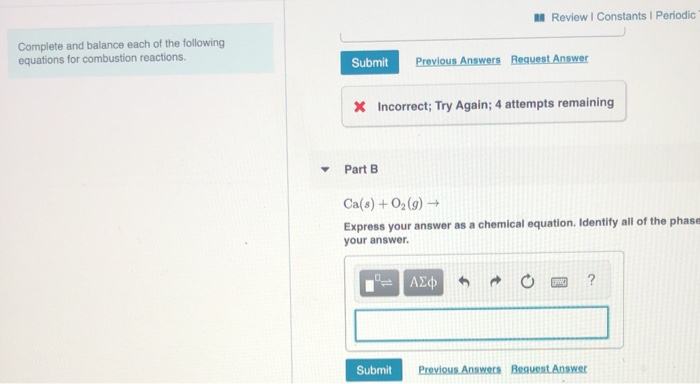A Review | Constants Periodic Complete and balance each of the following equations for combustion reactions. Submit Previous Answers Request Answer X Incorrect; Try Again; 4 attempts remaining Part B Ca(s) + 02(9) ► Express your answer as a chemical equation. Identify all of the phase your answer. Submit Previous. Answers Request Answer

• ### - Part A ReviewI Constants Periodic Table What are the H,O'] and [OH tor a solution with each of the following pH values? pH 3.2 Express your answers using one significant figure separated by a c...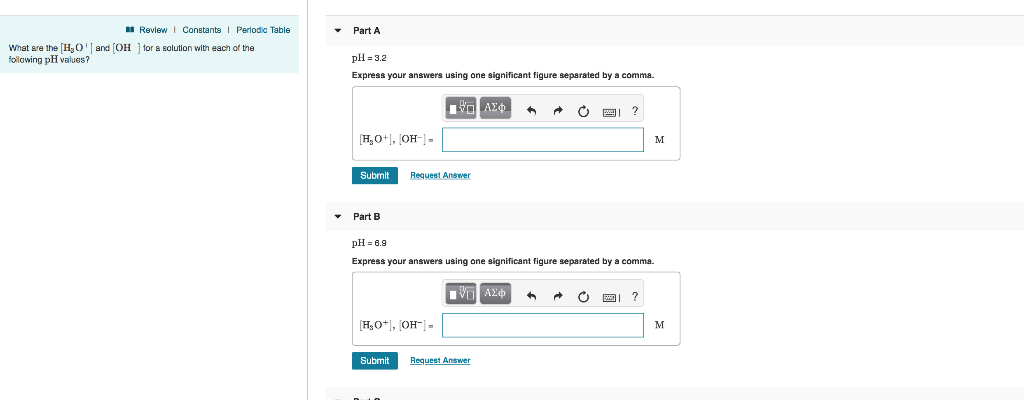- Part A ReviewI Constants Periodic Table What are the H,O'] and [OH tor a solution with each of the following pH values? pH 3.2 Express your answers using one significant figure separated by a comma. Submit Request Answer Part B pH 6.9 Express your answers using one significant figure separated by a comma. Submit Request Answer Review Constants Periodic Table What are the H O ' and [OH following pH values? for a solution with each of the Part...

• ### C Exercise 25.18 5 of 10 Review 1 Constants l Periodic Table Part A Bifocal glasses are used to c...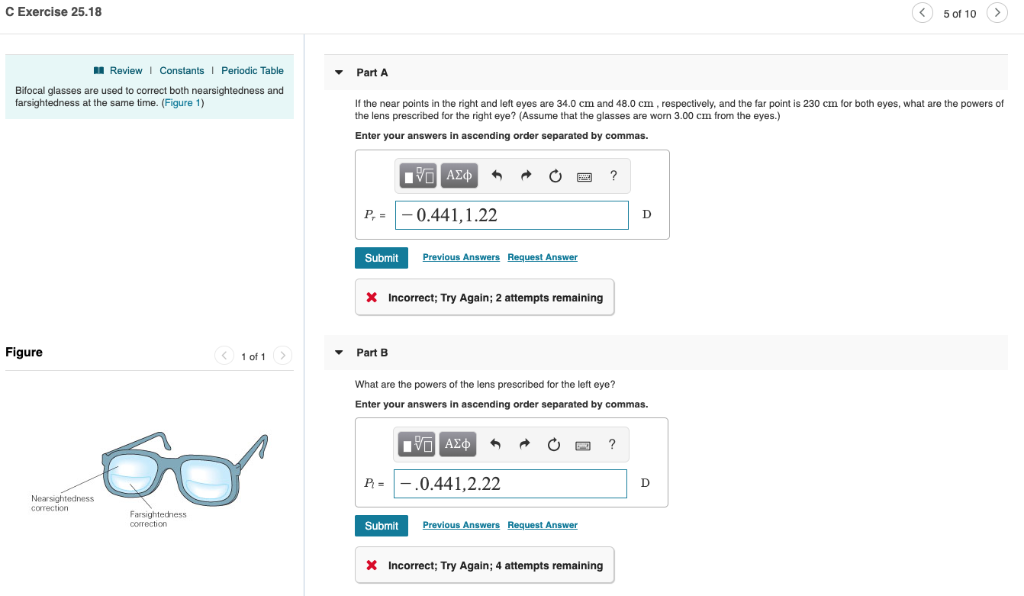C Exercise 25.18 5 of 10 Review 1 Constants l Periodic Table Part A Bifocal glasses are used to correct both nearsightedness and farsightedness at the same time. (Figure 1) If the near points in the right and left eyes are 34.0 cm and 48.0 cm, respectively, and the far point is 230 cm for both eyes, what are the powers of the lens prescribed for the right eye? (Assume that the glasses are worn 3.00 cm from the eyes.)...

• ### Complete the following table. [H3O+] [OH-] pH acidic, basic, or neutral? 1.3 x 10-'M 2.5 x...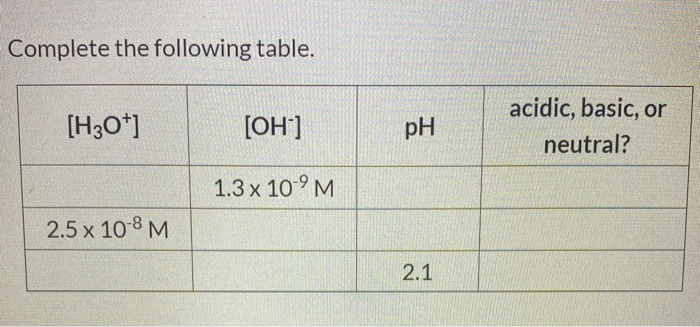Complete the following table. [H3O+] [OH-] pH acidic, basic, or neutral? 1.3 x 10-'M 2.5 x 10-8 M 2.1 Show transcribed image text

• ### Hon pil Adoras Part A Complete the first con Express your answers using two signatures. Enter...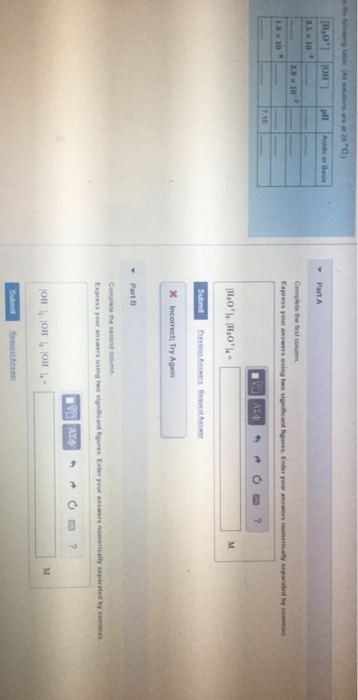Hon pil Adoras Part A Complete the first con Express your answers using two signatures. Enter your answers mencally para com VALPOO? [H.0bH90'l- Submit X Incorrect; Try Again Part 8 compare the second col Express your answering wo significant figures. Enter your answers mentally separated by com *VO AXO RO? HO | Hol - HO Submit [ H0 O PH Acidic or Basic Submit Nequest any 3.8 x 10 Part 1.8 x 10 Complete the third column Express your answers...

Free Homework App